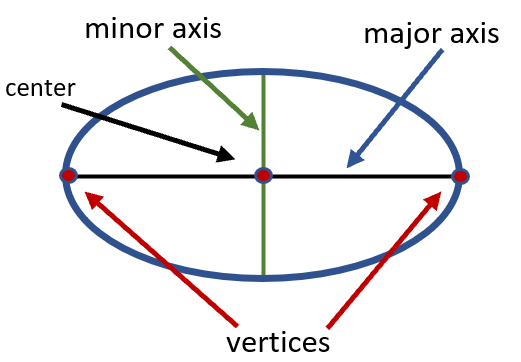# Math Worksheets Land

Math Worksheets For All Ages

# Math Worksheets Land

Math Worksheets For All Ages

Home > Grade Levels > High School Geometry >

# Ellipses Worksheets

There are several unique features that make each ellipse unique. It all starts with the center which is the point in the direct middle of the ellipse. This point helps us better understand the anatomy of the figure. Ellipses have measures that are of interest. A line that passes from the vertices to another vertices in the longest direction possible is called the major axis. The major axis is indicative of the longest part of the figure, the short part of the figure is called the minor axis. Again, this is indicated by a line passing through the center in the shortest direction. We will learn how to write the equation for ellipses and when constructing them we will need to remind ourselves of these parts that will help our creation come to life. These worksheets and lessons are really helpful for understanding the structure of an ellipse and how to map it.

### Aligned Standard: High School Geometry - HSG-GPE.A.3

• Answer Keys - These are for all the unlocked materials above.

### Homework Sheets

What is the equation of an figure after being given its vertices and foci?

• Homework 1 - To write the equation in standard form, the center, a2 and b2 must be found. The x-coordinate of the vertices and foci given are 0, so the center has to be (0,0).
• Homework 2 - |a| = length of semi-major axis, |b|= length of semi-minor axis
• Homework 3 - To find b2, use the foci equation. c is the distance from the center to the foci.

### Practice Worksheets

We want all of these figures to be in standard form.

• Practice 1 - Find the equations of an ellipse (in standard form) that has foci at (0,-9) and (0,9) and vertices at (0,-15) and (0,15).
• Practice 2 - Now that we have the values for b and c, we can substitute the values into the equation and simplify to find b.
• Practice 3 - Substitute 0 for h and k, the square root of 0 for b and 11 for a into the standard form equation.

### Math Skill Quizzes

The questions get a lot more mixed here.

• Quiz 1 - Write the standard equation of each figure. For example: 16x2 + 9y2 = 144
• Quiz 2 - Find the foci, vertices, and covertices of each ellipse: x2 /400 + y2/4 = 1
• Quiz 3 - There are basically three forms of problems here.

### How to Find the Equation of an EllipseBefore deriving an equation of an ellipse, here are a few important points that need to understand: A set of all points (x, y)(x ,y) in a plane such that the sum of distances from two fixed points is a constant, is known as Ellipse. Each fixed point is known as the "focus". Each ellipse has two axes of symmetry. The axis that is longer is known as the major axis, while the shorter axis is known as the minor axis. The endpoint of each major axis is known as the vertex, while the endpoints of the minor axis are considered the co-vertex. The midpoint of the major and minor axis is known as the center. Remember that the axes are perpendicular at the center of the figure. The foci are always situated on the major axis. The distance between the foci is less than the sum of the distances from the foci to any point on the ellipse.

For deriving the equation, the first step is the identification of the key information from the graph and place them in the pattern. For a horizontal ellipse, the pattern is : [(x - h)2 / a2] + [(y - k)2 / b2] = 1.

For a vertical ellipse, the pattern is: [(x - h)2 / b2] + [(y - k)2 / a2] = 1.

Here, (h, k) is the center point, a is the distance from the center point to the end of the major axis while b is the distance from the center point to the end of the major axis.

### The Importance of Ellipses in AstronomyThis geometric shape is one of the classical conic sections that can be formed by cutting a cone with a plane. It is one of the four common conic sections, the others being the circle, the hyperbola, and the parabola. The common version of the equation of this figure, as we studied in the above worksheets and lessons, is driven using Cartesian coordinates. The origin of this shape is located somewhere on the x-axis between the foci. The general nature of this figure is often described by its eccentricity which basically defines how un-circular something is. To help you relative understand this, a circle has zero eccentricity. A hyperbola has an eccentricity value greater than one. Ellipses generally fall somewhere between these two values. In astronomy these shapes are often used to describe and model the motions of celestial objects and their orbits that are dictated by gravity that is exerted by larger bodies. In our solar system (The Milky Way), the sun serves as the core focus for all orbits. In fact, Johannes Kepler's laws of planetary motion are based on the mathematical fundamentals of this shape. These laws of motion can be used to not only predict the orbital path of planets, but also asteroids and comets.

Unlock all the answers, worksheets, homework, tests and more!
Save Tons of Time! Make My Life Easier Now

## Thanks and Don't Forget To Tell Your Friends!

I would appreciate everyone letting me know if you find any errors. I'm getting a little older these days and my eyes are going. Please contact me, to let me know. I'll fix it ASAP.